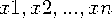Functions and CALL Routines

# EUCLID Function

Returns the Euclidean norm of the non-missing arguments.
 Category: Descriptive Statistics

## Syntax

 EUCLID(value-1 <,value-2 ...>)

### Arguments

value

specifies a numeric constant, variable, or expression.

If all arguments have missing values, then the result is a missing value. Otherwise, the result is the Euclidean norm of the non-missing values.

In the following example,are the values of the non-missing arguments.### Example 1: Calculating the Euclidean Norm of Non-missing Arguments

The following example returns the Euclidean norm of the non-missing arguments.

```data _null_;
x=euclid(.,3,0,.q,-4);
put x=;
run;```

SAS writes the following output to the log:

`x=5`

### Example 2: Calculating the Euclidean Norm When You Use a Variable List

The following example uses a variable list to calculate the Euclidean norm.

```data _null_;
x1 = 1;
x2 = 3;
x3 = 4;
x4 = 3;
x5 = 1;
x = euclid(of x1-x5);
put x=;
run;```

SAS writes the following output to the log:

`x=6`

 Functions:Previous Page | Next Page | Top of Page